``````library(ggplot2)
library(Sleuth3)
library(knitr)

# set some options to clean up output
opts_chunk\$set(message = FALSE, warning = FALSE)``````

# Anova assumptions

``qplot(reorder(spray, count), count, data = InsectSprays, geom= "boxplot")``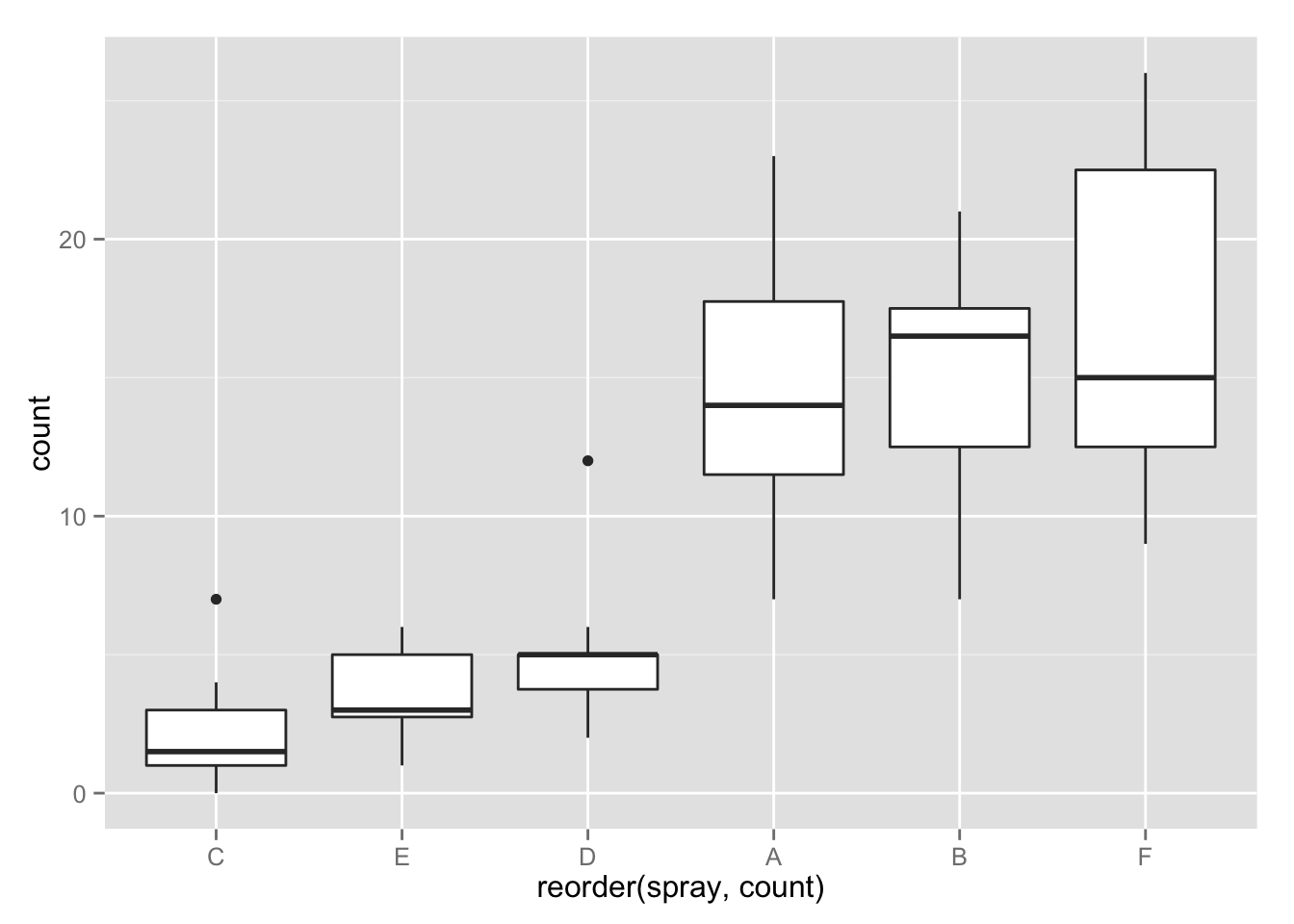## Do you see any evidence of violations of the ANOVA assumptions?

From the raw data we see some sprays (A, B and F) have much larger spread than the others. This is evidence that the maybe the assumption of equal population standard deviations is violated. Notice that these sprays also seen to have much higher centers. ## Take a look at the residual plots. Can you see the violations more easily?

``````spray_full <-  lm(count ~ spray, data = InsectSprays)
qplot(.fitted, .resid, data = spray_full) ``````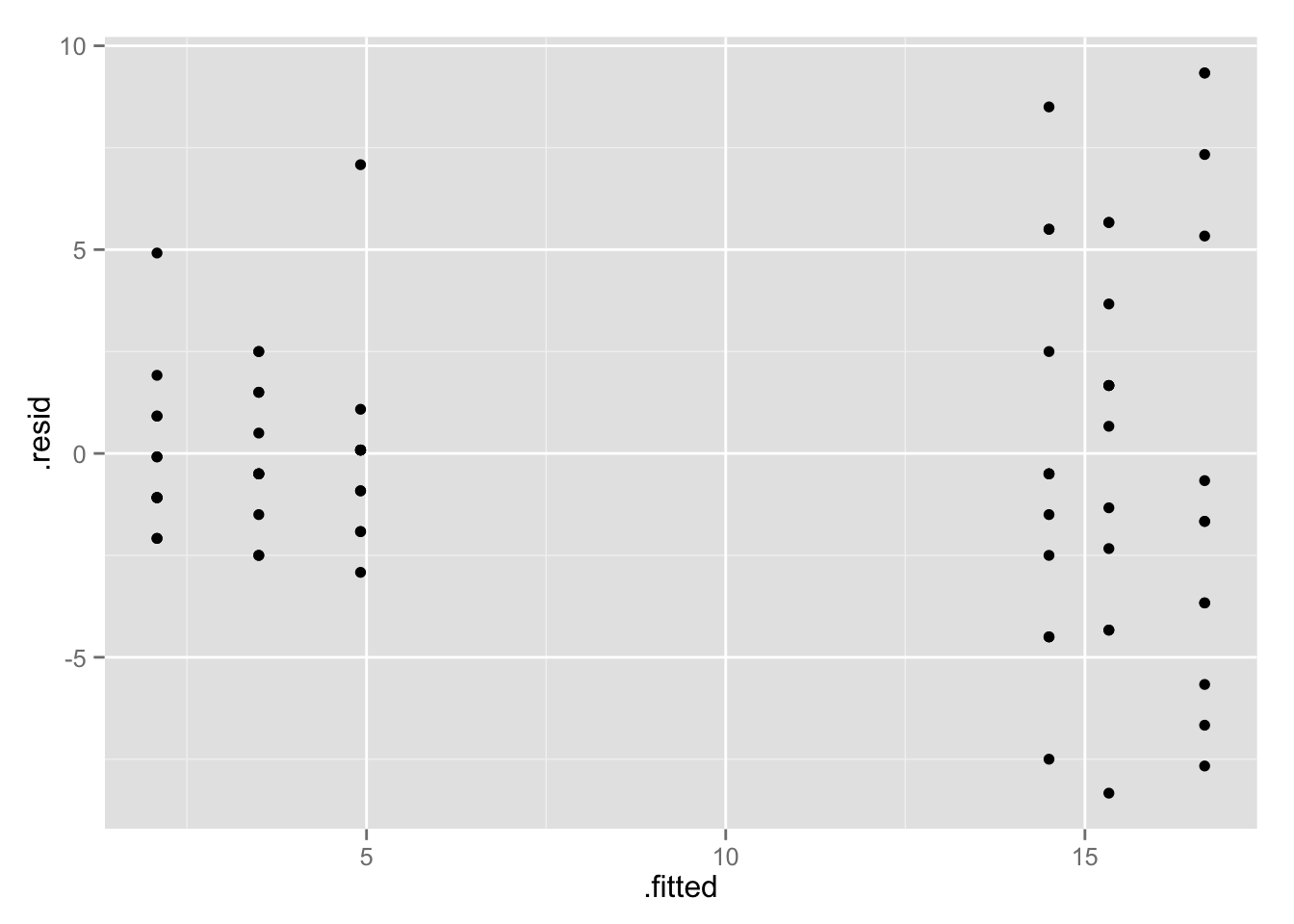In the plot of the residuals against the group averages (fitted values) we see a classic funnel shape: the spread of the residuals around zero gets larger as the group average gets larger. This is clear evidence the assumption of equal population standard deviations is violated. There is quite a bit of overplotting in the above plot, so a better plot might be to jitter the points:

``qplot(.fitted, .resid, data = spray_full, geom = "jitter" ) ``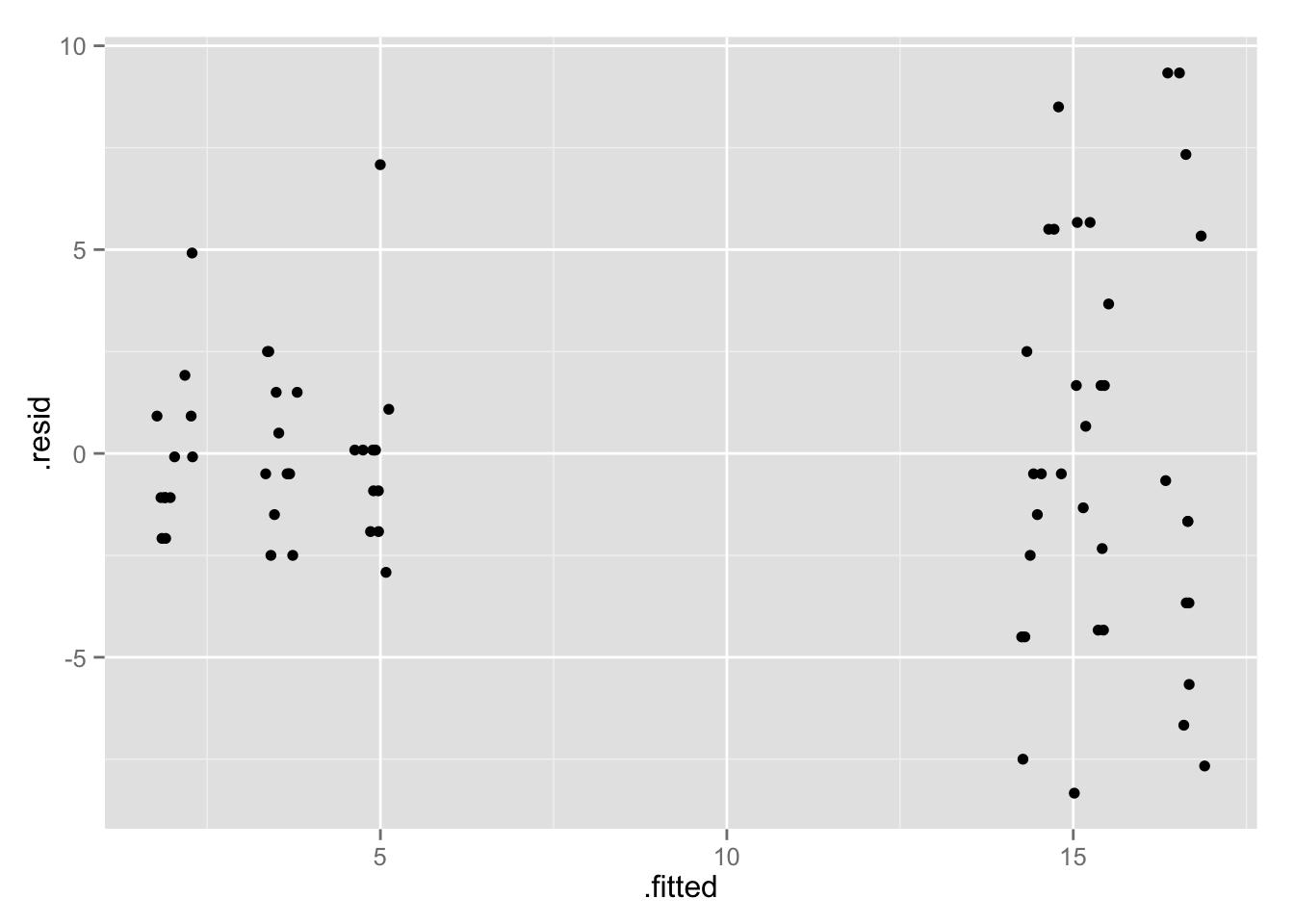``qplot(.fitted, .resid, data = spray_full, geom = "boxplot", group = spray) ``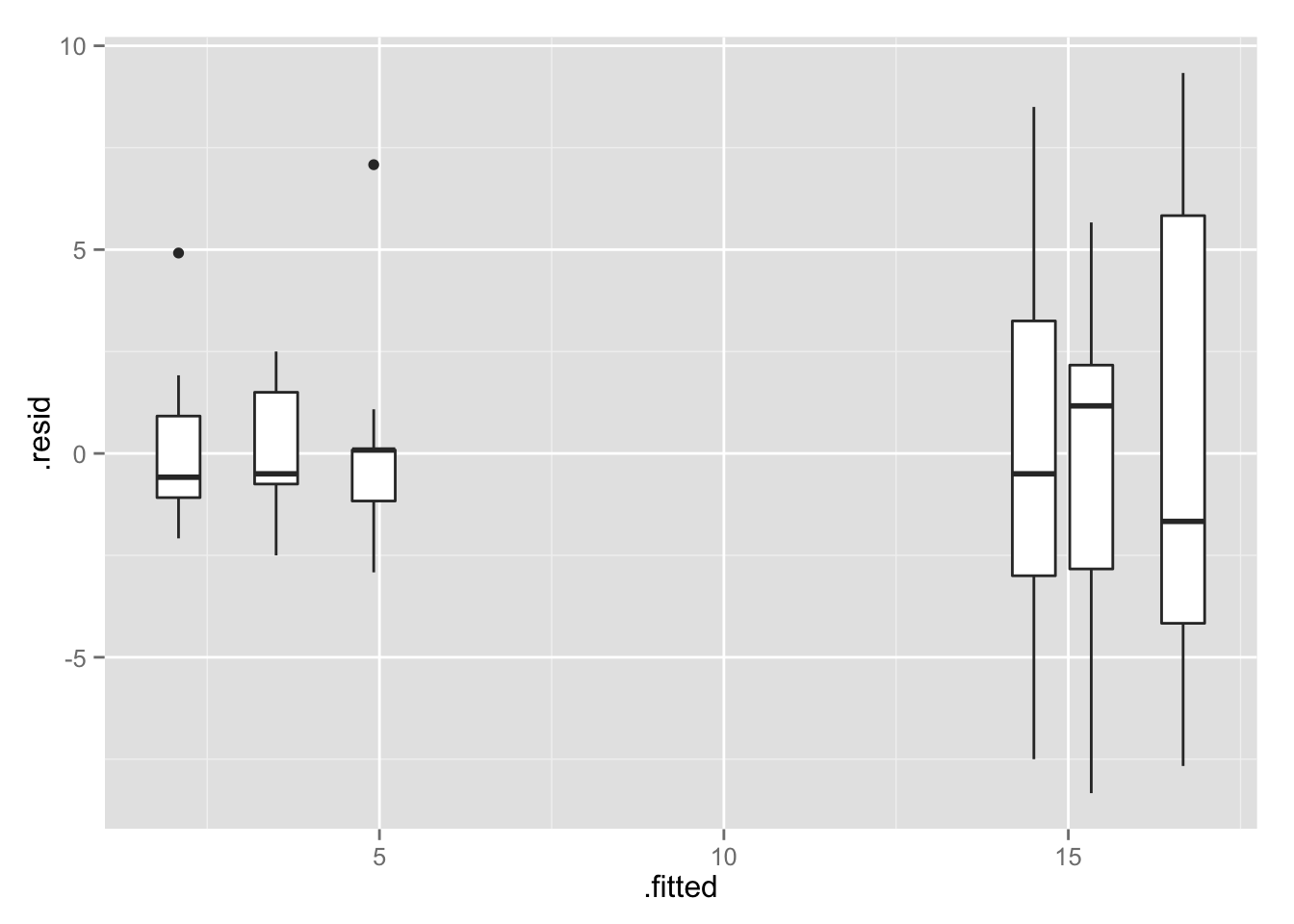``qplot(spray, .resid, data = spray_full )``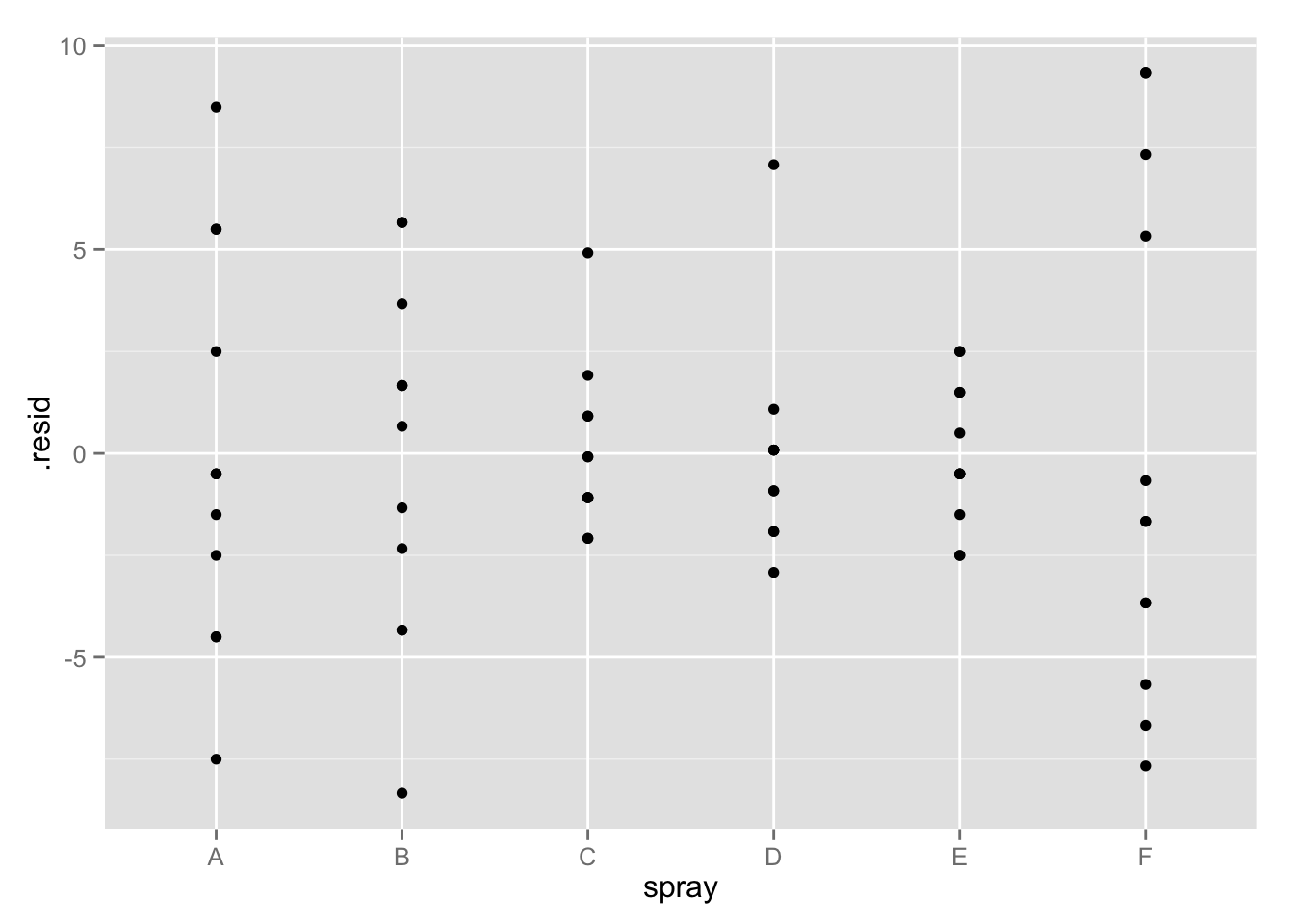The plot of the residuals against the groups reiterates that there is evidence the assumption of equal population standard deviations is violated. Now it’s clearer that is is groups C, D and E that have smaller spread compared to A, B and F. Again it might have been better to use boxplots:

``qplot(spray, .resid, data = spray_full, geom = "boxplot" )``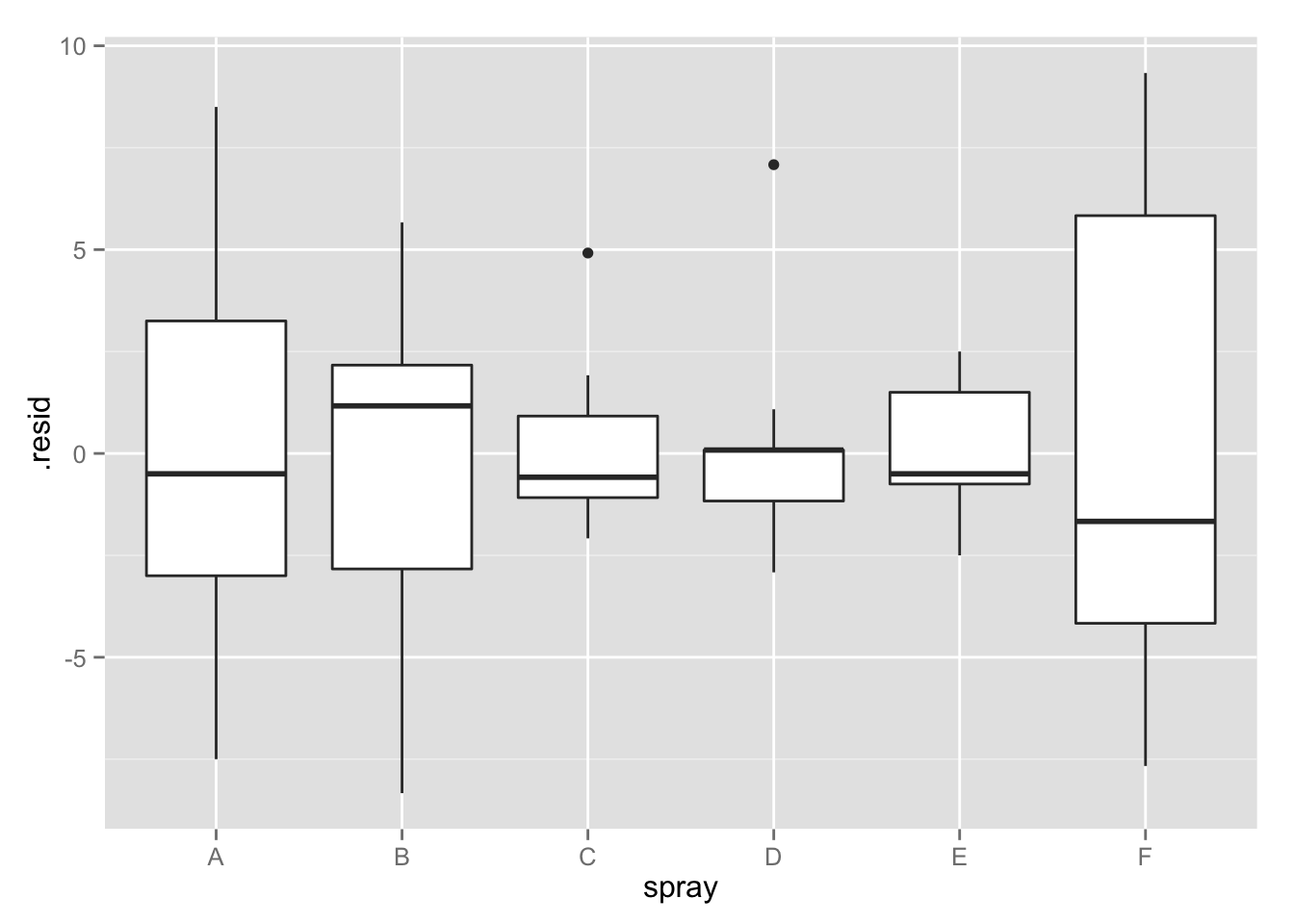``````source(url("http://stat511.cwick.co.nz/code/stat_qqline.r"))
qplot(sample = .resid, data = spray_full) + stat_qqline()``````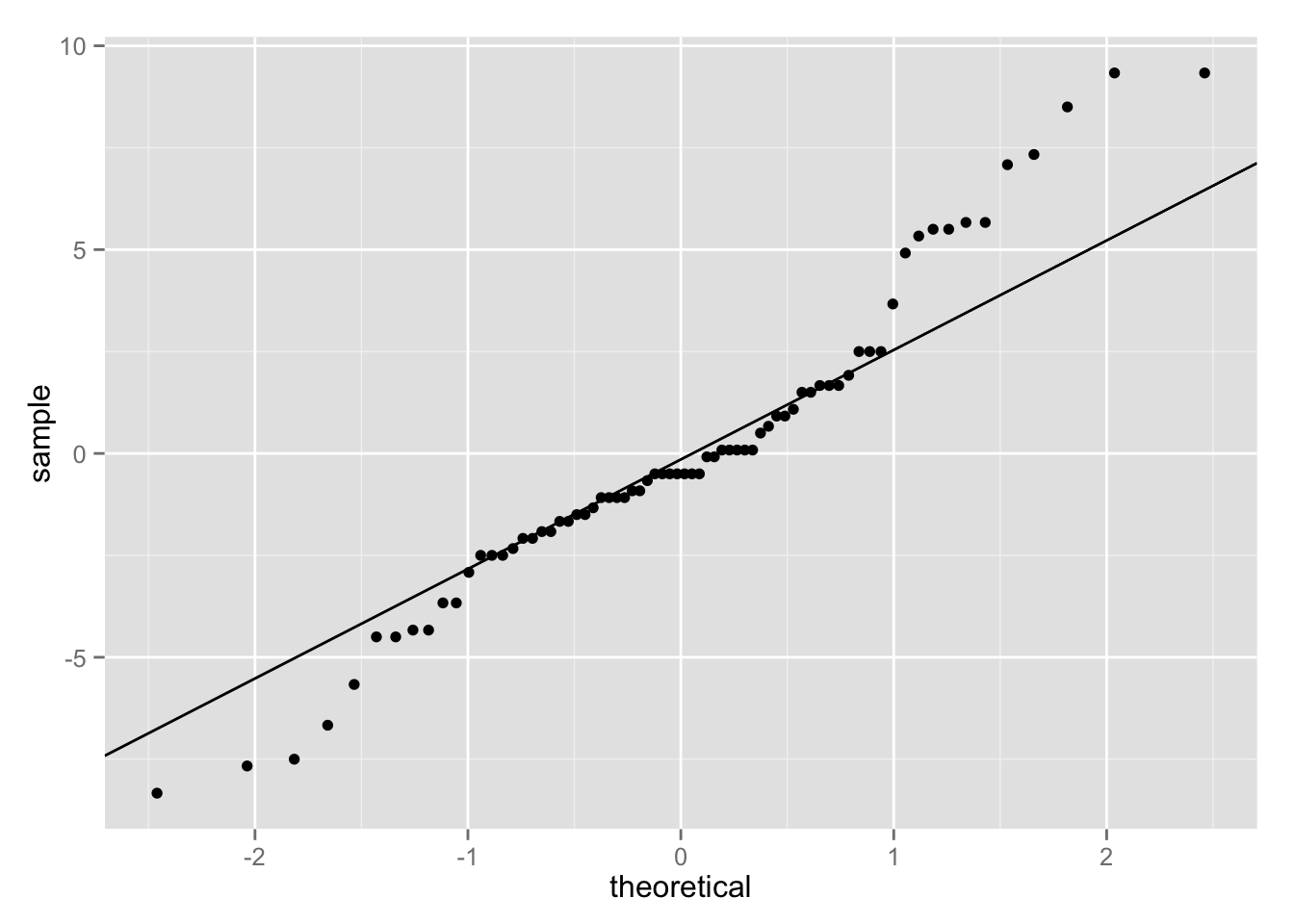The normal probability plot of the residuals shows some deviation from a straight line. The residuals seem to have longer tails that we would expect if the populations were Normally distributed. Be careful here! Part of the reason these residuals don’t look Normal, is that the population standard deviations aren’t equal. Generally, since Normality is less of a concern, we attempt to fix the non-equal SDs, before doing anything to fix non-Normality. ## Do the residual plots look better if we instead model the mean of the squareroot of insect count?

``````spray_full_sqrt <-  lm(sqrt(count) ~ spray, data = InsectSprays)
qplot(.fitted, .resid, data = spray_full_sqrt, geom = "jitter"  )``````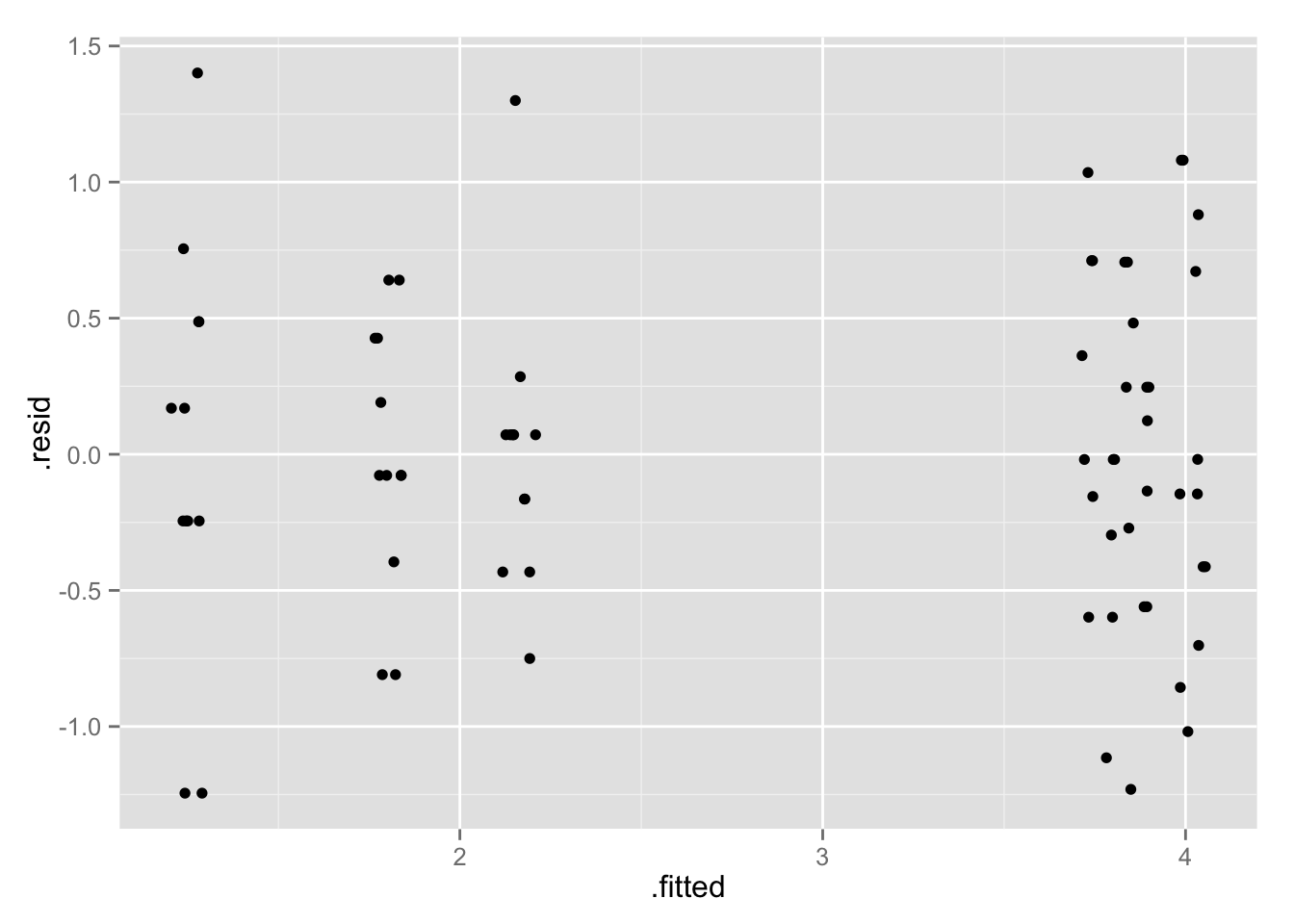Yes, big improvement! The funnel shape is gone in the residuals against fitted values plot.

``qplot(spray, .resid, data = spray_full_sqrt  )``